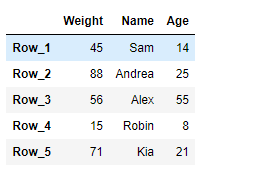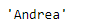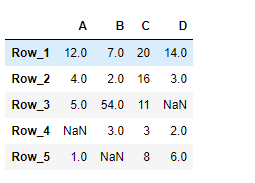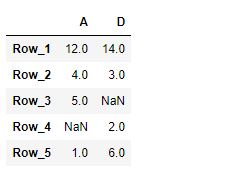Open in App
Not now

# Python | Pandas DataFrame.loc[]

• Difficulty Level : Basic
• Last Updated : 20 Feb, 2019

Pandas DataFrame is a two-dimensional size-mutable, potentially heterogeneous tabular data structure with labeled axes (rows and columns). Arithmetic operations align on both row and column labels. It can be thought of as a dict-like container for Series objects. This is the primary data structure of the Pandas.

Pandas` DataFrame.loc` attribute access a group of rows and columns by label(s) or a boolean array in the given DataFrame.

Syntax: DataFrame.loc

Parameter : None

Returns : Scalar, Series, DataFrame

Example #1: Use `DataFrame.loc` attribute to access a particular cell in the given Dataframe using the index and column labels.

 `# importing pandas as pd``import` `pandas as pd`` ` `# Creating the DataFrame``df ``=` `pd.DataFrame({``'Weight'``:[``45``, ``88``, ``56``, ``15``, ``71``],``                   ``'Name'``:[``'Sam'``, ``'Andrea'``, ``'Alex'``, ``'Robin'``, ``'Kia'``],``                   ``'Age'``:[``14``, ``25``, ``55``, ``8``, ``21``]})`` ` `# Create the index``index_ ``=` `[``'Row_1'``, ``'Row_2'``, ``'Row_3'``, ``'Row_4'``, ``'Row_5'``]`` ` `# Set the index``df.index ``=` `index_`` ` `# Print the DataFrame``print``(df)`

Output :Now we will use `DataFrame.loc` attribute to return the value present in the ‘Name’ column corresponding to the ‘Row_2’ label.

 `# return the value``result ``=` `df.loc[``'Row_2'``, ``'Name'``]`` ` `# Print the result``print``(result)`

Output :As we can see in the output, the `DataFrame.loc` attribute has successfully returned the value present at the desired location in the given DataFrame.

Example #2: Use `DataFrame.loc` attribute to return two of the column in the given Dataframe.

 `# importing pandas as pd``import` `pandas as pd`` ` `# Creating the DataFrame``df ``=` `pd.DataFrame({``"A"``:[``12``, ``4``, ``5``, ``None``, ``1``], ``                   ``"B"``:[``7``, ``2``, ``54``, ``3``, ``None``], ``                   ``"C"``:[``20``, ``16``, ``11``, ``3``, ``8``], ``                   ``"D"``:[``14``, ``3``, ``None``, ``2``, ``6``]}) `` ` `# Create the index``index_ ``=` `[``'Row_1'``, ``'Row_2'``, ``'Row_3'``, ``'Row_4'``, ``'Row_5'``]`` ` `# Set the index``df.index ``=` `index_`` ` `# Print the DataFrame``print``(df)`

Output :Now we will use `DataFrame.loc` attribute to return the values present in the ‘A’ and ‘D’ column of the Dataframe.

 `# return the values.``result ``=` `df.loc[:, [``'A'``, ``'D'``]]`` ` `# Print the result``print``(result)`

Output :As we can see in the output, the `DataFrame.loc` attribute has successfully returned the desired columns of the dataframe.

My Personal Notes arrow_drop_up